2. The Closed Model

The Closed Model is characterized by a positive curvature, k = 1. Thus, the spatial structure is that of the 3-sphere, similar to the surface of a sphere, but in 3 dimensions instead of 2. This model corresponds to a universe that begins at a `Big-Bang' and continues to expand until gravity finally halts the expansion. The universe will then collapse into a `Big-Crunch', which will resemble the reverse process of the `Big-Bang'. The ability of the matter (or energy) in the universe to halt the expansion obviously depends on the density. If the matter-energy density is too low, the universe will have enough momentum from the `bang' to escape the pull of gravity. In the Closed Model the density of the universe is great enough to halt the expansion and start a contraction. This corresponds to a value of> 1, which is evident from the use of the Friedmann equations with k = 1. Plugging this k value into the Friedmann equations (29), (28) and using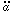= -qH2a one gets,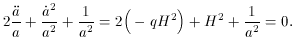This can be expressed as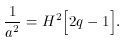(35)

Equation (28) takes the form,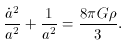(36)

Combining (35) and (36) gives,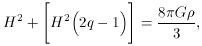or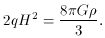Thus,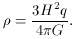(37)

Comparing (37) with the critical density (32) and the value of q > 1/2 in Table 2, it is evident that>c for the universe to be closed. In terms of, this gives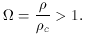(38)

The advantage of equation (37) above, is that the density is expressed all in quantities that can be measured. In that, if 2 of the 3 quantities are known the third may be found.